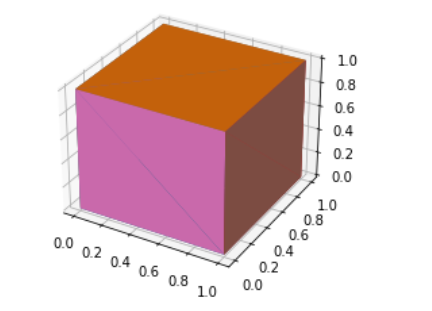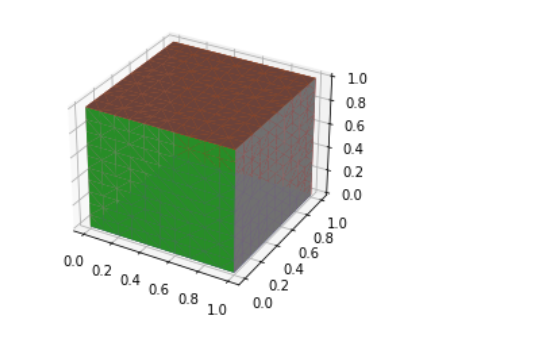2021-03-05 20:25

# 怎么用matplotlib用triangle mesh绘制长方体？

50

``````fig = plt.figure()
ax = fig.add_subplot(111, projection='3d')

x = np.random.rand(20)
y =  * 20
z =np.random.rand(20)

ax.plot_trisurf(x, y, z)
plt.show()``````
``````RuntimeError: Error in qhull Delaunay triangulation calculation: singular input data (exitcode=2); use python verbose option (-v) to see original qhull error.
``````
• 点赞
• 收藏
• 复制链接分享

#### 3条回答

• 总之就是，把你的 y =  * 20， 改成 y = np.arange(0,0.000001, 0.00000005)就行了

点赞 评论 复制链接分享
• plot_trisurf（）这个函数使用三角形填充曲面的，所以取的点数越多，效果越好，也可以每次只写出正方形四个顶点的坐标来绘制曲面

``````import numpy as np
import matplotlib.pyplot as plt

fig = plt.figure()
ax = fig.add_subplot(111, projection='3d')

x = [0,1,0,1]
y = [0,0,1,1]

z1 = [0,0.00000001,0.00000002,0.00000003] #不能绘制垂直地面的线，所以给他们加一个很小的数0.00000001
z2 = [1,1.00000001,1.00000002,1.00000003]

ax.plot_trisurf(x,y,z1)
ax.plot_trisurf(x,y,z2)

ax.plot_trisurf(z1,x,y)
ax.plot_trisurf(z2,x,y)

ax.plot_trisurf(z1,x,y)
ax.plot_trisurf(z2,x,y)

ax.plot_trisurf(x,z1,y)
ax.plot_trisurf(x,z2,y)

plt.show()``````

效果如图：点赞 评论 复制链接分享
• plot_trisurf（）这个函数的作用是，把一定数量的点（x,y,z），连接成一个平面，但不能垂直地面；

你的程序错误在于，y为常数，垂直地面所以报错，我的思路和你一样，绘制六个平面构成立方体，既然不能垂直地面（x,y不能是常数），那就给它加一个渐进的很小的数，就可以了，程序如下：

``````import numpy as np
import matplotlib.pyplot as plt

fig = plt.figure()
ax = fig.add_subplot(111, projection='3d')

x = np.arange(0,1.1,0.1)
y = np.arange(0,1.1,0.1)
xx,yy = np.meshgrid(x, y)

dz = np.arange(0,0.00000121,0.00000001) #xx,yy的数量为121，因此取121个很小的，渐进的数
dz = dz.reshape(xx.shape,xx.shape) # 保证xyz三个参数形状一样

z1 = np.zeros((xx.shape,xx.shape))
z2 = np.ones((xx.shape,xx.shape))

z1 = z1 + dz  #这样得到z1全都十分接近于0，但互相不相等
z2 = z2 + dz

ax.plot_trisurf(xx.flatten(), yy.flatten(), z1.flatten())
ax.plot_trisurf(xx.flatten(), yy.flatten(), z2.flatten())
ax.plot_trisurf(xx.flatten(), z1.flatten(), yy.flatten())
ax.plot_trisurf(xx.flatten(), z2.flatten(), yy.flatten())
ax.plot_trisurf(xx.flatten(), yy.flatten(), z1.flatten())
ax.plot_trisurf(xx.flatten(), yy.flatten(), z2.flatten())
ax.plot_trisurf(z1.flatten(), xx.flatten(), yy.flatten())
ax.plot_trisurf(z2.flatten(), xx.flatten(), yy.flatten())

plt.show()``````

程序运行结果如下：点赞 评论 复制链接分享